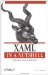# Vector

 Vector Vector is a structure and has no hierarchy.

`         <Vector             X="5"             Y="5" /> `

<Vector .../> represents the direction and magnitude of a line segment. It describes the movement of the x- and y-coordinates of a point along the x- and y-axes. If a line has start point (0,0) and end point (5,5), then the Vector describing the line segment is (5,5)essentially, the difference between the start and end points. Vector is a transformation mechanism; specifically, it is used to transform lines within a 2-D space.

#### Attributes

X (optional)

This Double value represents the x-component of the Vector. The default value is 0.

Y (optional)

This Double value represents the y-component of the Vector. The default value is 0.XAML in a Nutshell (In a Nutshell (OReilly))
ISBN: 0596526733
EAN: 2147483647
Year: 2007
Pages: 217